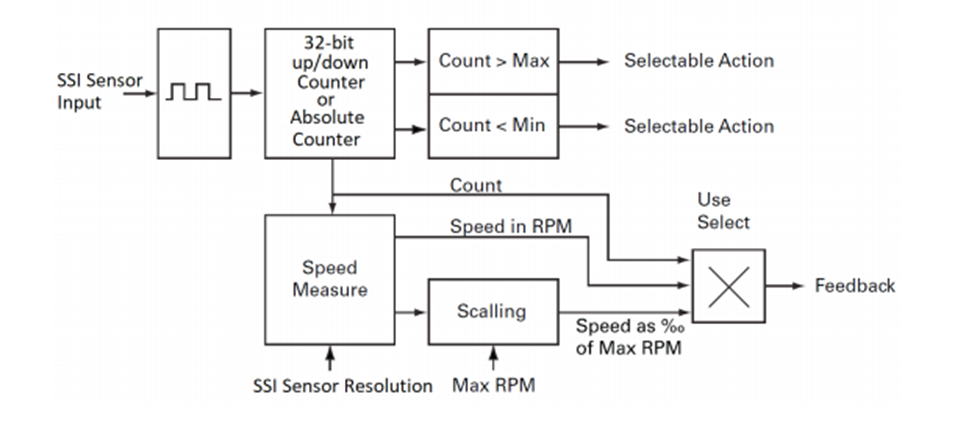Below are the basic steps required to run a motor in Open Loop by using its SSI sensors. Only the necessary information to run the motor will be provided, so for a more detailed explanations please refer to the Roboteq Controllers User Manual.

To run the motor in open loop with SSI follow the below steps:

1. Configure at General configurations the Molex Input to “SSI Sensors”2. Configure the number of “Pole Pairs”

Pole pairs parameter is essential for the sinusoidal commutation and for the speed calculation

3. Configure the switching mode to sinusoidal

SSI sensor can only be used for sinusoidal commutation

4. Configure the Reference seek power to the rated current of the motor

The reference seek power is the current that will be provided to the motor during the motor/sensor setup. If the reference current is too low or too high the motor/sensor setup might not be performed correctly5. Configure the sinusoidal angle sensor to SSI Sensor6. Configure the SSI sensor resolution

In case of single turn sensors, only the number of bits and the counter length must be configured. Typically, the single turn data will start at first bit and will have a length equal to the number of sensor bits. Therefore, "Number of bits" and "Counter number of bits" parameters should have the same value.In case of multi turn sensors, both signleturn and multiturn counters must be set. The start bit position of each counter must be located in the data frame and the data length must be defined. To help illustrate this, consider the following example: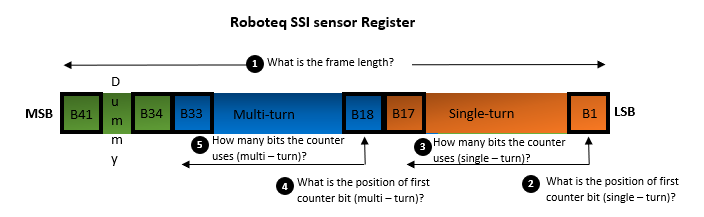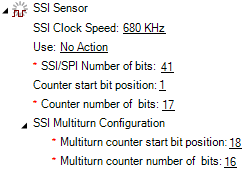7. Configure the FOC Flux and Torque gains

These gains concern the two PI controllers of the Current Loop, regulating the Torque and Flux current. The proper control of the Torque and Flux current is essential to achieve the optimal commutation and safe operation of the motor. The Current Loop can be tuned by knowing the Resistance and Inductance parameters of the motor. So there are two options:

Use the datasheet Parameters:

The datasheet parameters will give the phase-to-phase or the phase Resistance and Inductance values.

• If the parameters are phase-to-phase values they should be divided by 2
• All values should be changed to original units (Ohm and Henri and not mOhm and uH that are expressed usually)

Then the Flux and Torque gains can be calculated as follows:

Flux P gain = 314 * Phase Inductance (H)

Flux I gain = 314 * Phase Resistance (Ohm)

Torque P gain = 314 * Phase Inductance (H)

Torque I gain = 314 * Phase Resistance (Ohm)

Example:

p-p Resistance: 400 mOhm

p-p Inductance: 200 uH

Flux P gain = 314 * 0.000100 (H) = 0.0314

Flux I gain = 314 * 0.2 (Ohm) = 62.8

Torque P gain = 314 * 0.000100 (H) = 0.0314

Torque I gain =  314 * 0.2 (Ohm) = 62.8Tha value of 314 will give a fixed Bandwidth of 50 Hz. For more information about the FOC tuning refer to the Roboteq Controllers User Manual.

Use the Motor characterization Tool:

The Roboteq controller has a tool that can estimate the motor parameters and calculate the FOC values. This should be used only in case that the motor parameters are not known, becasue the estimated values might differ from the real.

To run the motor characterization go at diagnostics tab and click the motor characterization button. The motor will start making some noise.When asked select a Banwidth of 50 Hz. the controller will clalculate the FOC gains andautomatically save the FOC parameters.

8. Configure the Amp Limit parameter

The controller will prevent the current from exceeding the amp limit value by reducing its power. This parameter can be set equal to the peak current of the motor.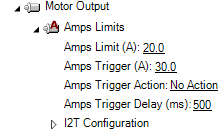9. Configure the Operating Mode to “Open Loop”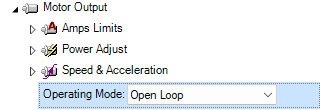10. Now go to the Diagnostics tab and run the Motor/Sensor Setup process.

The controller should turn for some degrees in CW and CCW direction by consuming a current that is equal to the reference seek power parameter. In the chart verify the following:

• The Sin parameter has the SSI angle. The angle value increase is linear and does not have any “steps”
• The Sensor Angle is almost linear
• The Motor Amps are equal to the Reference seek power11. Now the motor is ready to Run in Open Loop / Sinusoidal with SSI sensor!

To assess the performance of the motor, do the following:

• Turn the motor without load in both directions and verify that the motor current is not beyond the “no load current” and symmetrical in both directions. You can monitor the controller working parameters on the Run tab of the Roborun+ Utility.
• Verify that the Motor flux amps parameter has a small fluctuation and is kept close to zero.
• Verify that the FOC angle correction parameter is stable or that it stabilizes in satisfying time by changing the motor load/speed.
• Verify that the Internal Sensor RPM parameter has a positive value by giving a positive motor command. If the measured speed is reverse, you can fix it by inverting the pole pairs parameter sign. Note that is important to have a speed of the correct sign, otherwise the motor will not be controller properly in Closed Loop.